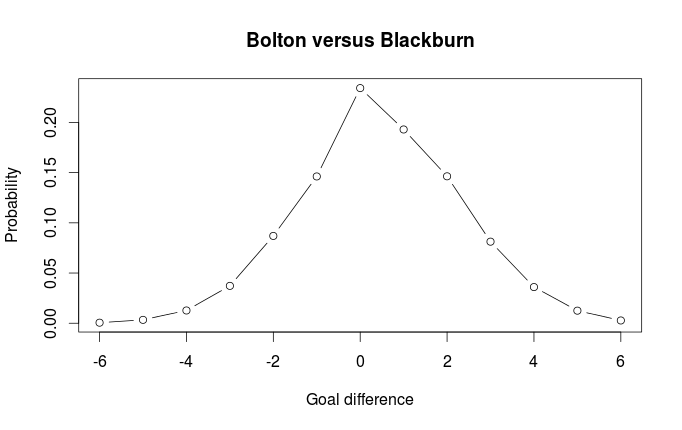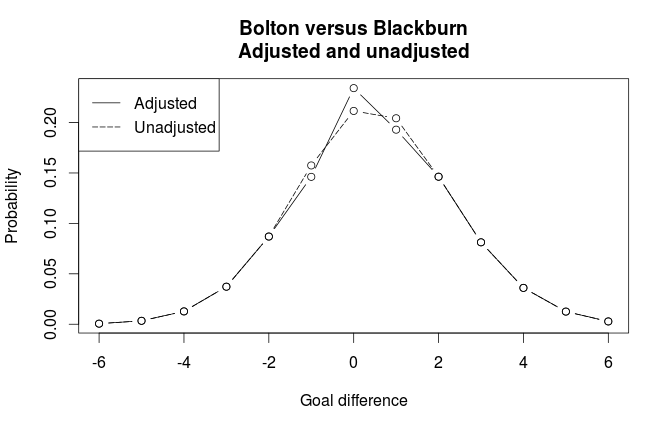The Dixon-Coles model for predicting football matches in R (part 2)

Part 1 ended with running the optimizer function to estimate the parameters in the model:

library(alabama)
res <- auglag(par=par.inits, fn=DCoptimFn, heq=DCattackConstr, DCm=dcm)

# Take a look at the parameters
res\$par

In part 1 I fitted the model to data from the 2011-12 Premier League season. Now it’s time to use the model to make a prediction. As an example I will predict the result of Bolton playing at home against Blackburn.

The first thing we need to do is to calculate the mu and lambda parameters, which is (approximately anyway) the expected number of goals scored by the home and away team. To do this wee need to extract the correct parameters from the res\$par vector. Recall that I in the last post gave the parameters informative names that consists of the team name prefixed by either Attack or Defence.
Also notice that I have to multiply the team parameters and then exponentiate the result to get the correct answer.

Update: For some reason I got the idea that the team parameters should be multiplied together, instead of added together, but I have now fixed the code and the results.

# Expected goals home
lambda <- exp(res\$par['HOME'] + res\$par['Attack.Bolton'] + res\$par['Defence.Blackburn'])

# Expected goals away
mu <- exp(res\$par['Attack.Blackburn'] + res\$par['Defence.Bolton'])

We get that Bolton is expected to score 2.07 goals and Blackburn is expected to score 1.59 goals.

Since the model assumes dependencies between the number of goals scored by the two teams, it is insufficient to just plug the lambda and mu parameters into R’s built-in Poisson function to get the probabilities for the number of goals scored by the two teams. We also need to incorporate the adjustment for the low-scoring results as well. One strategy to do this is to first create a matrix based on the simple independent Poisson distributions:

maxgoal <- 6 # will be useful later
probability_matrix <- dpois(0:maxgoal, lambda) %*% t(dpois(0:maxgoal, mu))

The number of home goals follows the vertical axis and the away goals follow the horizontal.

Now we can use the estimated dependency parameter rho to create a 2-by-2 matrix with scaling factors, that is then element-wise multiplied with the top left elements of the matrix calculated above:

Update: Thanks to Mike who pointed out a mistake in this code.

scaling_matrix <- matrix(tau(c(0,1,0,1), c(0,0,1,1), lambda, mu, res\$par['RHO']), nrow=2)
probability_matrix[1:2, 1:2] <- probability_matrix[1:2, 1:2] * scaling_matrix

With this matrix it is easy to calculate the probabilities for the three match outcomes:

HomeWinProbability <- sum(probability_matrix[lower.tri(probability_matrix)])
DrawProbability <- sum(diag(probability_matrix))
AwayWinProbability <- sum(probability_matrix[upper.tri(probability_matrix)])

This gives a probability of 0.49 for home win, 0.21 for draw and 0.29 for away win.

Calculating the probabilities for the different goal differences is a bit trickier. The probabilities for each goal difference can be found by adding up the numbers on the diagonals, with the sum of the main diagonal being the probability of a draw.

awayG <- numeric(maxgoal)
for (gg in 2:maxgoal){
awayG[gg-1] <- sum(diag(probability_matrix[,gg:(maxgoal+1)]))
}
awayG[maxgoal] <- probability_matrix[1,(maxgoal+1)]

homeG <- numeric(maxgoal)
for (gg in 2:maxgoal){
homeG[gg-1] <- sum(diag(probability_matrix[gg:(maxgoal+1),]))
}
homeG[maxgoal] <- probability_matrix[(maxgoal+1),1]

goaldiffs <- c(rev(awayG), sum(diag(probability_matrix)), homeG)
names(goaldiffs) <- -maxgoal:maxgoal

It is always nice to plot the probability distribution:We can also see compare this distribution with the distribution without the Dixon-Coles adjustment (i.e. the goals scored by the two teams are independent):As expected, we see that the adjustment gives higher probability for draw, and lower probabilities for goal differences of one goal.

The Dixon-Coles model for predicting football matches in R (part 1)

Please have a look at the improved code for this model that I have posted here.

When it comes to Poisson regression models for football results, the 1997 paper Modelling Association Football Scores and Inefficiencies in the Football Betting Market (pdf) by Dixon and Coles is often mentioned. In this paper the authors describe an improvement of the independent goals model. The improvement consists of modeling a dependence between the probabilities for the number of goals less than 2 for both teams. They also improve the model by incorporating a time perspective, so that matches played a long time a go does not have as much influence on the parameter estimates.

The model by Dixon and Coles is not as easy to fit as the independent Poisson model I have described earlier. There is no built-in function in R that can estimate it’s parameters, and the authors provide little details about how to implement it. Mostly as an exercise, I have implemented the model in R, but without the time down-weighting scheme.

The estimating procedure uses a technique called maximum likelihood. This is perhaps the most commonly used method for estimating parameters in statistical models. The way it works is that you specify a way to calculate the likelihood of your data for a given set of parameters, and then you need to find the set of parameters that gives the highest possible likelihood of your data. The independent Poisson model is also fitted using a maximum likelihood method. The difference here is that the likelihood used by Dixon and Coles is non-standard.

The model is pretty much similar to other regression models I have discussed. Each team has an attack and a defense parameter, and from a function of these the expected number of goals for each team in a match is calculated. For the rest of this post I am going to assume you have read the paper. There is a link to it in the first paragraph.

The most obvious thing we have to do is to implement the function referred to by the greek letter Tau. This is the function that, dependent on the Rho parameter, computes the degree in which the probabilities for the low scoring goals changes.

tau <- Vectorize(function(xx, yy, lambda, mu, rho){
if (xx == 0 & yy == 0){return(1 - (lambda*mu*rho))
} else if (xx == 0 & yy == 1){return(1 + (lambda*rho))
} else if (xx == 1 & yy == 0){return(1 + (mu*rho))
} else if (xx == 1 & yy == 1){return(1 - rho)
} else {return(1)}
})

We can now make a function for the likelihood of the data. A common trick when implementing likelihood functions is to use the log-likelihood instead. The reason is that when the probabilities for each data point for a given set of parameters are multiplied together, they will be too small for the computer to handle. When the probabilities are log-transformed you can instead just add them together.

What this function does is that it takes the vectors of mu (expected home goals) and lambda (expected away goals), Rho, and the vectors of observed home and away goals, and computes the log-likelihood for all the data.

DClogLik <- function(y1, y2, lambda, mu, rho=0){
#rho=0, independence
#y1: home goals
#y2: away goals
sum(log(tau(y1, y2, lambda, mu, rho)) + log(dpois(y1, lambda)) + log(dpois(y2, mu)))
}

The team specific attack and defense parameters are not included in the log-likelihood function. Neither is the code that calculates the expected number of goals for each team in a match (lambda and mu). Before we can calculate these for each match, we need to do some data wrangling. Here is a function that takes a data.frame formated like the data from football-data.co.uk, and returns a list with design matrices and vectors with the match results.

DCmodelData <- function(df){

hm <- model.matrix(~ HomeTeam - 1, data=df, contrasts.arg=list(HomeTeam='contr.treatment'))
am <- model.matrix(~ AwayTeam -1, data=df)

team.names <- unique(c(levels(df\$HomeTeam), levels(df\$AwayTeam)))

return(list(
homeTeamDM=hm,
awayTeamDM=am,
homeGoals=df\$FTHG,
awayGoals=df\$FTAG,
teams=team.names
))
}

Now we create a function that calculates the log-likelihod from a set of parameters and the data we have. First it calculates the values for lambda and mu for each match, then it passes these and the number of goals scored in each match to the log-likelihood function.

This function needs to be written in such a way that it can be used by another function that will find the parameters that maximizes the log-likelihood. First, all the parameters needs to be given to a single argument in the form of a vector (the params argument). Also, the log-likelihood is multiplied by -1, since the optimization function we are going to use only minimizes, but we want to maximize.

DCoptimFn <- function(params, DCm){

home.p <- params
rho.p <- params

nteams <- length(DCm\$teams)
attack.p <- matrix(params[3:(nteams+2)], ncol=1)
defence.p <- matrix(params[(nteams+3):length(params)], ncol=1)

lambda <- exp(DCm\$homeTeamDM %*% attack.p + DCm\$awayTeamDM %*% defence.p + home.p)
mu <- exp(DCm\$awayTeamDM %*% attack.p + DCm\$homeTeamDM %*% defence.p)

return(
DClogLik(y1=DCm\$homeGoals, y2=DCm\$awayGoals, lambda, mu, rho.p) * -1
)
}

One more thing we need before we start optimizing is a function that helps the optimizer handle the constraint that all the attack parameters must sum to 1. If this constraint isn’t given, it will be impossible to find a unique set of parameters that maximizes the likelihood.

DCattackConstr <- function(params, DCm, ...){
nteams <- length(DCm\$teams)
attack.p <- matrix(params[3:(nteams+2)], ncol=1)
return((sum(attack.p) / nteams) - 1)
}

Now we are finally ready to find the parameters that maximizes the likelihood based on our data. First, load the data (in this case data from the 2011-12 premier league), and properly handle it with our DCmodelData function:

dcm <- DCmodelData(dta)

Now we need to give a set of initial estimates of our parameters. It is not so important what specific values these are, but should preferably be in the same order of magnitude as what we think the estimated parameters should be. I set all attack parameters to 0.1 and all defense parameters to -0.8.

#initial parameter estimates
attack.params <- rep(.01, times=nlevels(dta\$HomeTeam))
defence.params <- rep(-0.08, times=nlevels(dta\$HomeTeam))
home.param <- 0.06
rho.init <- 0.03
par.inits <- c(home.param, rho.init, attack.params, defence.params)
#it is also usefull to give the parameters some informative names
names(par.inits) <- c('HOME', 'RHO', paste('Attack', dcm\$teams, sep='.'), paste('Defence', dcm\$teams, sep='.'))

To optimize with equality constraints (all attack parameters must sum to 1) we can use the auglag function in the alabama package. This takes about 40 seconds to run on my laptop, much longer than the independent Poisson model fitted with the built in glm function. This is because the auglag function uses some general purpose algorithms that can work with a whole range of home-made functions, while the glm function is implemented with a specific set of models in mind.

library(alabama)
res <- auglag(par=par.inits, fn=DCoptimFn, heq=DCattackConstr, DCm=dcm)

Voilà! Now the parameters can be found by the command res\$par. In a follow-up post I will show how we can use the model to make prediction of match outcomes.

Team Attack Defence
Arsenal 1.37 -0.91
Aston Villa 0.69 -0.85
Blackburn 0.94 -0.47
Bolton 0.92 -0.48
Chelsea 1.23 -0.97
Everton 0.94 -1.15
Fulham 0.93 -0.89
Liverpool 0.89 -1.13
Man City 1.56 -1.43
Man United 1.52 -1.31
Newcastle 1.10 -0.88
Norwich 1.02 -0.62
QPR 0.82 -0.65
Stoke 0.64 -0.87
Sunderland 0.86 -0.99
Swansea 0.85 -0.89
Tottenham 1.24 -1.09
West Brom 0.86 -0.88
Wigan 0.81 -0.71
Wolves 0.79 -0.42
Home 0.27
Rho -0.13